# Quadratic Equations: Definition, Formula And Examples

Table of Contents

## Quadratic Equations

Quadratic equations are one of the most important topics asked in various competitive exams such as Banking, SSC and others. Quadratic equations are generally simple to answer only if one follows a proper approach and formula. We are providing you with the Quadratic equations definition, formula and questions with answers to help you understand the concept in a better way. You can study from the notes provided below and get acquainted with the important tricks.

Click here for SSC CGL Tier 2 Study material

## Quadratic Equations: Definition

Quadratic equations can be defined by the name “Quad” which means “Square” In a quadratic equation, one of the variables is squared. This type of equation is also called “Equation of degree 2”.

An algebraic expression of the form: ax² + bx + c = 0, where a ≠ 0, b, c ∈ R is called a quadratic equation.

The solutions of a quadratic equation can be found by equating to 0. The solutions are called ‘roots’ or ‘zeroes’.

### Roots of the Quadratic Equations

A root of the quadratic equation ax² + bc + c = 0 is a number α (real or complex) such that a a²+ba+c=0 then (x-a) is factor of ax²+bx+c. The roots of the quadratic equation are
x=(-b±√(b²-4ac))/2a

### Quadratic Equations: Nature of Roots

The value of x at which value of the equation will be zero.
Roots are imaginary: b² – 4ac ≤ 0
Roots are real: b² – 4ac ≥ 0

### Sum & product of roots

Let there are two roots named α & β, then,
a=(-b+√(b²-4ac))/2a  & β=(-b-√(b²-4ac))/2a
Sum of root: α + β = (-b)/a
Product of root: αβ = c/a

### Quadratic Equations: Examples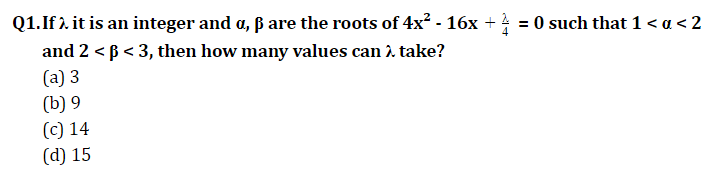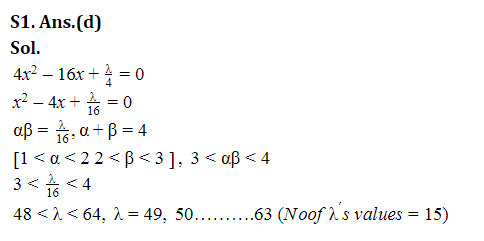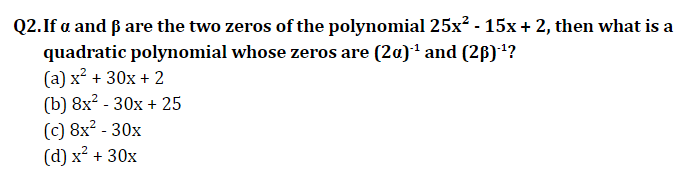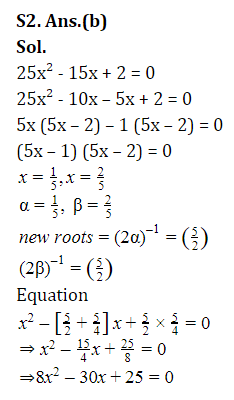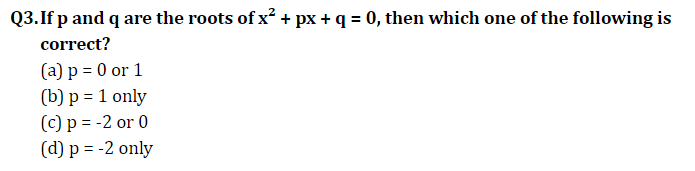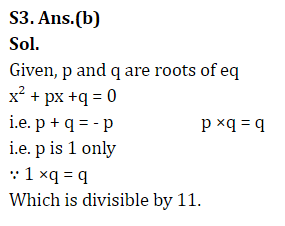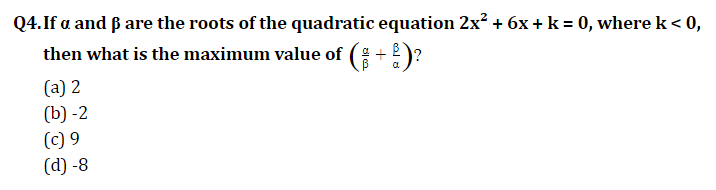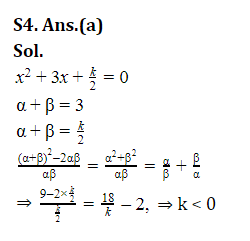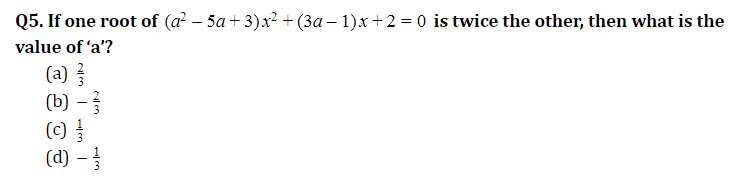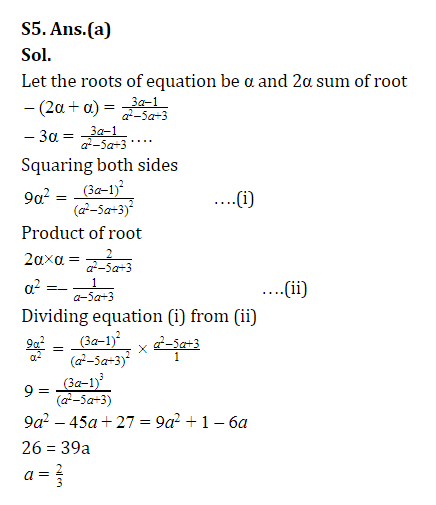### Click here for more Maths Study Notes

Preparing for SSC Exams in 2020? Register now to get free study material

×

## Download success!

Thanks for downloading the guide. For similar guides, free study material, quizzes, videos and job alerts you can download the Adda247 app from play store.

Thank You, Your details have been submitted we will get back to you.

## Leave a commentJoin India's largest learning destination

What You Will get ?

•Job Alerts
•Daily Quizes
•Subject-Wise Quizes
•Current Affairs
•Previous year question papers
•Doubt Solving session

## Login

OR

Forgot Password?Join India's largest learning destination

What You Will get ?

•Job Alerts
•Daily Quizes
•Subject-Wise Quizes
•Current Affairs
•Previous year question papers
•Doubt Solving session

## Sign Up

ORJoin India's largest learning destination

What You Will get ?

•Job Alerts
•Daily Quizes
•Subject-Wise Quizes
•Current Affairs
•Previous year question papers
•Doubt Solving session

## Forgot Password

Enter the email address associated with your account, and we'll email you an OTP to verify it's you.Join India's largest learning destination

What You Will get ?

•Job Alerts
•Daily Quizes
•Subject-Wise Quizes
•Current Affairs
•Previous year question papers
•Doubt Solving session

## Enter OTP

Please enter the OTP sent to
/6

Did not recive OTP?

Resend in 60sJoin India's largest learning destination

What You Will get ?

•Job Alerts
•Daily Quizes
•Subject-Wise Quizes
•Current Affairs
•Previous year question papers
•Doubt Solving session

## Change PasswordJoin India's largest learning destination

What You Will get ?

•Job Alerts
•Daily Quizes
•Subject-Wise Quizes
•Current Affairs
•Previous year question papers
•Doubt Solving session

## Almost there

Please enter your phone no. to proceed
+91Join India's largest learning destination

What You Will get ?

•Job Alerts
•Daily Quizes
•Subject-Wise Quizes
•Current Affairs
•Previous year question papers
•Doubt Solving session

## Enter OTP

Please enter the OTP sent to Edit Number

Did not recive OTP?

Resend 60

## By skipping this step you will not recieve any free content avalaible on adda247, also you will miss onto notification and job alerts

Are you sure you want to skip this step?

## By skipping this step you will not recieve any free content avalaible on adda247, also you will miss onto notification and job alerts

Are you sure you want to skip this step?